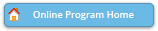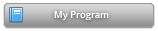#### Abstract Details

 Activity Number: 103 - New Developments on Statistical Machine Learning Type: Invited Date/Time: Monday, July 29, 2019 : 8:30 AM to 10:20 AM Sponsor: IMS Abstract #300506 Title: Statistical and Computational Guarantees of EM with Random Initialization Author(s): Harrison H. Zhou* and Yihong Wu Companies: Yale Uinversity and Yale University Keywords: EM ; Gaussian Mixtures; Estimation; Clustering; Optimality ; Convergence Abstract: This talk considers parameter estimation in the two-component symmetric Gaussian mixtures in $d$ dimensions with $n$ independent samples. We show that, even in the absence of any separation between components, with high probability, the EM algorithm converges to an estimate in at most $O(\sqrt{n} \log n)$ iterations, which is within $O((d/n)^{1/4} (\log n)^{3/4})$ in Euclidean distance to the true parameter, provided that $n=\Omega(d \log^2 d)$. This is within a logarithmic factor to the minimax optimal rate of $(d/n)^{1/4}$. The proof relies on establishing (a) a non-linear contraction behavior of the population EM mapping (b) concentration of the EM trajectory near the population version, to prove that random initialization works. This is in contrast to previous analysis in Daskalakis, Tzamos, and Zampetakis (2017) that requires sample splitting and restart the EM iteration after normalization, and Balakrishnan, Wainwright, and Yu (2017) that requires strong conditions on both the separation of the components and the quality of the initialization. Furthermore, we obtain the asymptotic efficient estimation when the signal is stronger than the minimax rate.

Authors who are presenting talks have a * after their name.